您可以捐助，支持我们的公益事业。 1元 10元 50元 认证码：必填求知 文章 文库 Lib 视频 iProcess 课程 认证 咨询 工具 讲座 Modeler Code要资料订阅捐助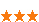1290 次浏览     评价： 好 中 差
2018-8-20

 编辑推荐: 本文来自于csdn，介绍了CUDA编程模型基础，向量加法实例，矩阵乘法实例等。

2006年，NVIDIA公司发布了CUDA（http://docs.nvidia.com/cuda/），CUDA是建立在NVIDIA的CPUs上的一个通用并行计算平台和编程模型，基于CUDA编程可以利用GPUs的并行计算引擎来更加高效地解决比较复杂的计算难题。近年来，GPU最成功的一个应用就是深度学习领域，基于GPU的并行计算已经成为训练深度学习模型的标配。目前，最新的CUDA版本为CUDA 9。

GPU并不是一个独立运行的计算平台，而需要与CPU协同工作，可以看成是CPU的协处理器，因此当我们在说GPU并行计算时，其实是指的基于CPU+GPU的异构计算架构。在异构计算架构中，GPU与CPU通过PCIe总线连接在一起来协同工作，CPU所在位置称为为主机端（host），而GPU所在位置称为设备端（device），如下图所示。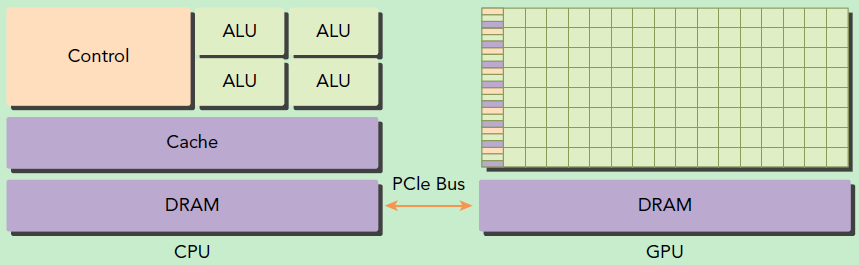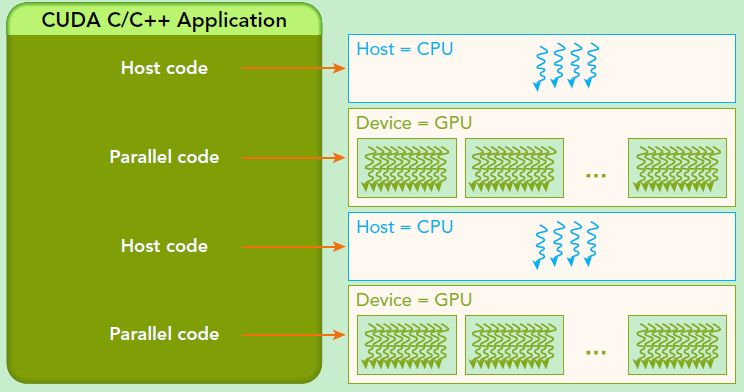CUDA是NVIDIA公司所开发的GPU编程模型，它提供了GPU编程的简易接口，基于CUDA编程可以构建基于GPU计算的应用程序。CUDA提供了对其它编程语言的支持，如C/C++，Python，Fortran等语言，这里我们选择CUDA C/C++接口对CUDA编程进行讲解。开发平台为Windows 10 + VS 2013，Windows系统下的CUDA安装教程可以参考这里http: //docs.nvidia.com /cuda / cuda-installation-guide- microsoft-windows/index.html

1 CUDA编程模型基础

1.分配host内存，并进行数据初始化；

2.分配device内存，并从host将数据拷贝到device上；

3.调用CUDA的核函数在device上完成指定的运算；

4.将device上的运算结果拷贝到host上；

5.释放device和host上分配的内存。

__global__：在device上执行，从host中调用（一些特定的GPU也可以从device上调用），返回类型必须是void，不支持可变参数参数，不能成为类成员函数。注意用__global__定义的kernel是异步的，这意味着host不会等待kernel执行完就执行下一步。

__device__：在device上执行，仅可以从device中调用，不可以和__global__同时用。

__host__：在host上执行，仅可以从host上调用，一般省略不写，不可以和__global__同时用，但可和__ device __，此时函数会在device和host都编译。

 dim3 grid(3, 2); dim3 block(4, 3); kernel_fun<<< grid, block >>>(prams...);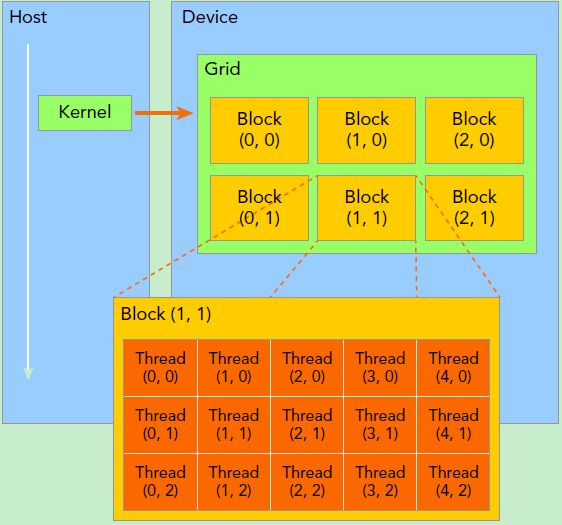threadIdx.x = 1 threadIdx.y = 1 blockIdx.x = 1 blockIdx.y = 1

kernel的这种线程组织结构天然适合vector,matrix等运算，如我们将利用上图2-dim结构实现两个矩阵的加法，每个线程负责处理每个位置的两个元素相加，代码如下所示。线程块大小为(16, 16)，然后将N*N大小的矩阵均分为不同的线程块来执行加法运算。

 // Kernel定义 __global__ void MatAdd (float A[N][N], float B[N][N], float C[N][N]) { int i = blockIdx.x * blockDim.x + threadIdx.x; int j = blockIdx.y * blockDim.y + threadIdx.y; if (i < N && j < N) C[i][j] = A[i][j] + B[i][j]; } int main() { ... // Kernel 线程配置 dim3 threadsPerBlock(16, 16); dim3 numBlocks (N / threadsPerBlock.x, N / threadsPerBlock .y); // kernel调用 MatAdd <<>>(A, B, C); ... }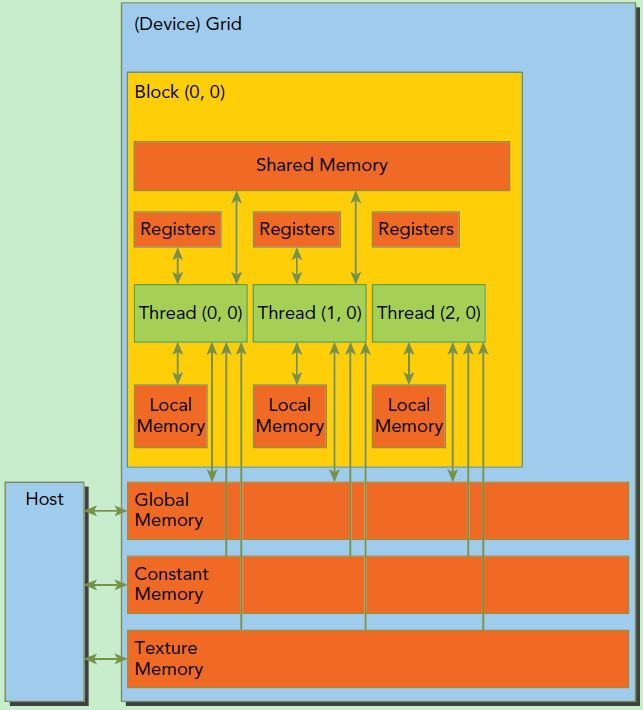CUDA内存模型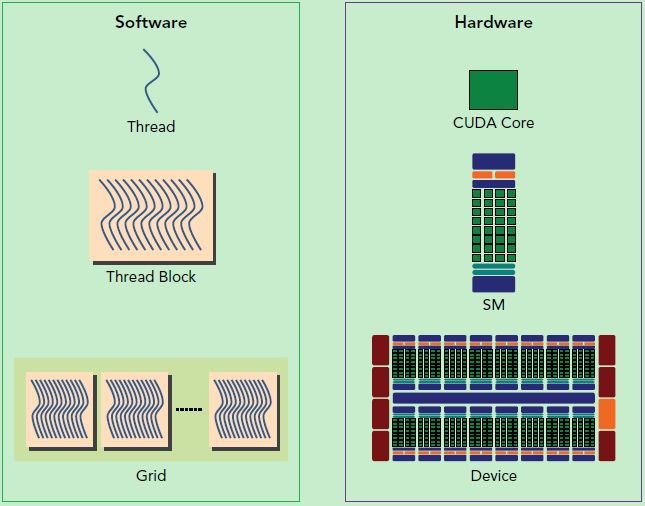CUDA编程的逻辑层和物理层

 int dev = 0; cudaDeviceProp devProp; CHECK (cudaGetDeviceProperties (&devProp, dev)); std : :cout << "使用GPU device " << dev << ": " << devProp .name << std::endl; std::cout << "SM的数量：" << devProp.multiProcessorCount << std::endl; std::cout << "每个线程块的共享内存大小：" << devProp.sharedMemPerBlock / 1024.0 << " KB" << std ::endl; std ::cout << "每个线程块的最大线程数：" << devProp .maxThreadsPerBlock << std::endl; std ::cout << "每个EM的最大线程数：" << devProp . maxThreadsPerMultiProcessor << std::endl; std ::cout << "每个EM的最大线程束数：" << devProp.maxThreadsPerMultiProcessor / 32 << std::endl; // 输出如下 使用GPU device 0: GeForce GT 730 SM的数量：2 每个线程块的共享内存大小：48 KB 每个线程块的最大线程数：1024 每个EM的最大线程数：2048 每个EM的最大线程束数：64

2 向量加法实例

 cudaError_t cudaMalloc(void** devPtr, size_t size);

 cudaError_t cudaMalloc(void** devPtr, size_t size);

cudaMemcpyDeviceToHost 及cudaMemcpyDeviceToDevice，如 cudaMemcpyHostToDevice将host 上数据拷贝到device上。

 // 两个向量加法kernel，grid和block均为一维 __global__ void add(float* x, float * y, float* z, int n) { // 获取全局索引 int index = threadIdx.x + blockIdx.x * blockDim.x; // 步长 int stride = blockDim.x * gridDim.x; for (int i = index; i < n; i += stride) { z[i] = x[i] + y[i]; } }

 int main() { int N = 1 << 20; int nBytes = N * sizeof(float); // 申请host内存 float *x, *y, *z; x = (float*)malloc(nBytes); y = (float*)malloc(nBytes); z = (float*)malloc(nBytes); // 初始化数据 for (int i = 0; i < N; ++i) { x[i] = 10.0; y[i] = 20.0; } // 申请device内存 float *d_x, *d_y, *d_z; cudaMalloc((void**)&d_x, nBytes); cudaMalloc((void**)&d_y, nBytes); cudaMalloc((void**)&d_z, nBytes); // 将host数据拷贝到device cudaMemcpy ((void*)d_x, (void*)x, nBytes, cudaMemcpyHostToDevice ); cudaMemcpy ((void*)d_y, (void*)y, nBytes, cudaMemcpyHostToDevice); // 定义kernel的执行配置 dim3 blockSize(256); dim3 gridSize((N + blockSize.x - 1) / blockSize.x); // 执行kernel add << < gridSize, blockSize >> >(d_x, d_y, d_z, N); // 将device得到的结果拷贝到host cudaMemcpy((void*)z, (void*)d_z, nBytes, cudaMemcpyHostToDevice); // 检查执行结果 float maxError = 0.0; for (int i = 0; i < N; i++) maxError = fmax(maxError, fabs(z[i] - 30.0)); std::cout << "最大误差: " << maxError << std::endl; // 释放device内存 cudaFree(d_x); cudaFree(d_y); cudaFree(d_z); // 释放host内存 free(x); free(y); free(z); return 0; }

kernel的线程层级结构如下图所示：

kernel的线程层次结构. 来源：https://devblogs.nvidia.com/even-easier-introduction-cuda/

 nvprof cuda9.exe ==7244== NVPROF is profiling process 7244, command: cuda9.exe 最大误差: 4.31602e+008 ==7244== Profiling application: cuda9.exe ==7244== Profiling result: Type Time(%) Time Calls Avg Min Max Name GPU activities: 67.57% 3.2256ms 2 1.6128ms 1.6017ms 1.6239ms [CUDA memcpy HtoD] 32.43% 1.5478ms 1 1.5478ms 1.5478ms 1.5478ms add(float*, float*, float*, int)

 cudaError_t cudaMallocManaged (void **devPtr, size_t size, unsigned int flag=0);

 int main() { int N = 1 << 20; int nBytes = N * sizeof(float); // 申请托管内存 float *x, *y, *z; cudaMallocManaged ((void**)&x, nBytes); cudaMallocManaged ((void**)&y, nBytes); cudaMallocManaged ((void**)&z, nBytes); // 初始化数据 for (int i = 0; i < N; ++i) { x[i] = 10.0; y[i] = 20.0; } // 定义kernel的执行配置 dim3 blockSize (256); dim3 gridSize ((N + blockSize.x - 1) / blockSize.x); // 执行kernel add << < gridSize, blockSize >> >(x, y, z, N); // 同步device 保证结果能正确访问 cudaDeviceSynchronize(); // 检查执行结果 float maxError = 0.0; for (int i = 0; i < N; i++) maxError = fmax(maxError, fabs(z[i] - 30.0)); std::cout << "最大误差: " << maxError << std::endl; // 释放内存 cudaFree(x); cudaFree(y); cudaFree(z); return 0; }

3 矩阵乘法实例

 // 矩阵类型，行优先，M(row, col) = *(M.elements + row * M.width + col) struct Matrix { int width; int height; float *elements; Matrix(int w, int h, float* e = NULL) { width = w; height = h; elements = e; } };

 // 获取矩阵A的(row, col)元素 __device__ float getElement(const Matrix A, int row, int col) { return A.elements[row * A.width + col]; } // 为矩阵A的(row, col)元素赋值 __device__ void setElement(Matrix A, int row, int col, float value) { A.elements[row * A.width + col] = value; } // 矩阵相乘kernel，2-D，每个线程计算一个元素 __global__ void matMulKernel(const Matrix A, const Matrix B, Matrix C) { float Cvalue = 0.0; int row = threadIdx.y + blockIdx.y * blockDim.y; int col = threadIdx.x + blockIdx.x * blockDim.x; for (int i = 0; i < A.width; ++i) { Cvalue += getElement(A, row, i) * getElement(B, i, col); } setElement(C, row, col, Cvalue); }

 int main() { int width = 1 << 10; int height = 1 << 10; Matrix A(width, height, NULL); Matrix B(width, height, NULL); Matrix C(width, height, NULL); int nBytes = width * height * sizeof(float); // 申请托管内存 cudaMallocManaged ((void**)&A.elements, nBytes); cudaMallocManaged ((void**)&B.elements, nBytes); cudaMallocManaged ((void**)&C.elements, nBytes); // 初始化数据 for (int i = 0; i < width * height; ++i) { A.elements[i] = 1.0; B.elements[i] = 2.0; } // 定义kernel的执行配置 dim3 blockSize (32, 32); dim3 gridSize ((width + blockSize.x - 1) / blockSize . x , (height + blockSize.y - 1) / blockSize.y); // 执行kernel matMulKernel << < gridSize, blockSize >> >(A, B, C); // 同步device 保证结果能正确访问 cudaDeviceSynchronize(); // 检查执行结果 float maxError = 0.0; for (int i = 0; i < width * height; i++) maxError = fmax(maxError, fabs(C.elements[i] - 2 * width)); std::cout << C.elements << std::endl; std::cout << "最大误差: " << maxError << std::endl; return 0; }

 nvprof cuda9.exe ==2456== NVPROF is profiling process 2456, command: cuda9.exe 最大误差: 0 ==2456== Profiling application: cuda9.exe ==2456== Profiling result: Type Time(%) Time Calls Avg Min Max Name GPU activities: 100.00% 2.67533s 1 2.67533s 2.67533s 2.67533s matMulKernel(Matrix, Matrix, Matrix) API calls: 92.22% 2.67547s 1 2.67547s 2.67547s 2.67547s cudaDeviceSynchronize 6.06% 175.92ms 3 58.640ms 2.3933ms 170.97ms cudaMallocManaged 1.65% 47.845ms 1 47.845ms 47.845ms 47.845ms cudaLaunch 0.05% 1.4405ms 94 15.324us 0ns 938.54us cuDeviceGetAttribute 0.01% 371.49us 1 371.49us 371.49us 371.49us cuDeviceGetName 0.00% 13.474us 1 13.474us 13.474us 13.474us cuDeviceTotalMem 0.00% 6.9300us 1 6.9300us 6.9300us 6.9300us cudaConfigureCall 0.00% 3.8500us 3 1.2830us 385ns 1.9250us cuDeviceGetCount 0.00% 3.4650us 3 1.1550us 0ns 2.3100us cudaSetupArgument 0.00% 2.3100us 2 1.1550us 385ns 1.9250us cuDeviceGet ==2456== Unified Memory profiling result: Device "GeForce GT 730 (0)" Count Avg Size Min Size Max Size Total Size Total Time Name 2048 4.0000KB 4.0000KB 4.0000KB 8.000000MB 22.70431ms Host To Device 266 46.195KB 32.000KB 1.0000MB 12.00000MB 7.213048ms Device To Host1290 次浏览  评价: 好 中 差订阅捐助
 相关文章 云计算的架构 对云计算服务模型 云计算核心技术剖析 了解云计算的漏洞
 相关文档 云计算简介 云计算简介与云安全 下一代网络计算--云计算 软浅析云计算
 相关课程 云计算原理与应用 云计算应用与开发 CMMI体系与实践 基于CMMI标准的软件质量保证